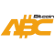Bitcoin ABC  0.22.13 P2P Digital Currency
tests_impl.h File Reference

Go to the source code of this file.

## Functions

static int recovery_test_nonce_function (unsigned char *nonce32, const unsigned char *msg32, const unsigned char *key32, const unsigned char *algo16, void *data, unsigned int counter)

void test_ecdsa_recovery_api (void)

void test_ecdsa_recovery_end_to_end (void)

void test_ecdsa_recovery_edge_cases (void)

void run_recovery_tests (void)

## ◆ recovery_test_nonce_function()

 static int recovery_test_nonce_function ( unsigned char * nonce32, const unsigned char * msg32, const unsigned char * key32, const unsigned char * algo16, void * data, unsigned int counter )
static

Definition at line 10 of file tests_impl.h.

Here is the call graph for this function:
Here is the caller graph for this function:

## ◆ run_recovery_tests()

 void run_recovery_tests ( void )

Definition at line 381 of file tests_impl.h.

Here is the call graph for this function:
Here is the caller graph for this function:

## ◆ test_ecdsa_recovery_api()

 void test_ecdsa_recovery_api ( void )

Definition at line 31 of file tests_impl.h.

Here is the call graph for this function:
Here is the caller graph for this function:

## ◆ test_ecdsa_recovery_edge_cases()

 void test_ecdsa_recovery_edge_cases ( void )

Definition at line 208 of file tests_impl.h.

Here is the call graph for this function:
Here is the caller graph for this function:

## ◆ test_ecdsa_recovery_end_to_end()

 void test_ecdsa_recovery_end_to_end ( void )

Definition at line 150 of file tests_impl.h.

Here is the call graph for this function:
Here is the caller graph for this function: